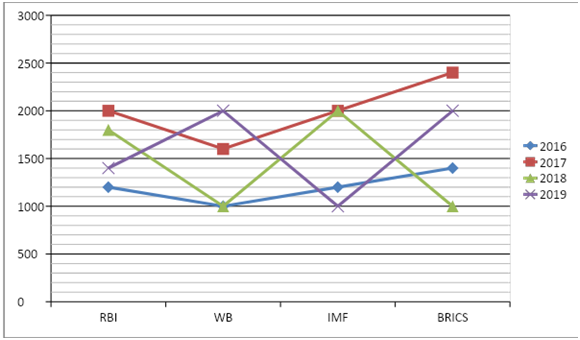# SBI Clerk Prelims 2021 Quantitative Aptitude Questions (Day-11)

Dear Aspirants, Our IBPS Guide team is providing new series of Quantitative Aptitude Questions for SBI Clerk Prelims 2020 so the aspirants can practice it on a daily basis. These questions are framed by our skilled experts after understanding your needs thoroughly. Aspirants can practice these new series questions daily to familiarize with the exact exam pattern and make your preparation effective.

Start Quiz

Directions (1-5): Find out the wrong number in the following number series.

1) 8, 15, 28, 53, 102, 210

A.15

B.28

C.53

D.102

E.210

2) 15, 40, 76, 125, 190, 270

A.40

B.76

C.125

D.190

E.270

3) 20, 75, 192, 579, 1740, 5223

A.75

B.192

C.579

D.1740

E.5223

4) 80, 20, 10, 11, 12, 14.4

A.20

B.10

C.11

D.12

E.14.4

5)
10, 11, 15, 20, 40, 65

A.11

B.15

C.20

D.40

E.65

Directions (6-10):
Study the following information carefully and answer the questions given below.

The given line graph shows the number of meetings held by four different organization in four years6) The number of meetings held in 2016 in all the organization together is what percent of the number of meetings held in 2017 in all the organization together?

A.40%

B.50%

C.60%

D.70%

E.30%

7) What is the ratio of the number of BRICS meeting held in 2019 and 2017 together to the number of IMF meeting held in 2016 and 2018 together?

A.7:4

B.8:5

C.9:5

D.10:9

E.11:8

8) What is the difference between the total number of WB meeting held in all years together and the number of BRICS meeting held in all the years together?

A.1200

B.1400

C.800

D.1000

E.1500

9) The number of RBI meeting held in 2018 is approximately what percent of the total number of RBI meeting held in all the years together?

A.22%

B.28%

C.30%

D.32%

E.26%

10) What is the difference between the number of meeting held in all the organization together in 2018 and 2019?

A.400

B.600

C.300

D.800

E.500

8 * 2 – 1= 15

15 * 2 – 2 = 28

28 * 2 – 3 = 53

53 * 2 – 4 = 102

102 * 2 – 5 = 199 (not 210)

15 + 52 = 40

40 + 62 = 76

76 + 72 = 125

125 + 82 = 189 (not 190)

189 + 92 = 270

20 * 3 + 3 = 63 (not 75)

63 * 3 + 3 = 192

192 * 3 + 3 = 579

579 * 3 + 3 = 1740

1740 * 3 + 3 = 5223

80 ÷ 5 + 4 = 20

20 ÷ 5 + 6 = 10

10 ÷ 5 + 8 = 10 (not 11)

10 ÷ 5 + 10 = 12

12 ÷ 5 + 12 = 14.4

10 + 12 = 11

11 + 22 = 15

15 + 32 = 24 (not 20)

24 + 42 = 40

40 + 52 = 65

Required percentage = (1200 + 1000 + 1200 + 1400)/(2000 + 1600 + 2000 + 2400) * 100

= (4800/8000) * 100

= 60%

Required ratio = (2000 + 2400):(1200 + 2000)

= 4400:3200

= 11:8

Required difference = (1400 + 2400 + 1000 + 2000) – (1000 + 1600 + 1000 + 2000)

= 6800 – 5600

= 1200

Required percentage = 1800/(1200 + 2000 + 1800 + 1400) * 100

= (1800/6400) * 100

= 28%

Required difference = (1400 + 2000 + 1000 + 2000) – (1800 + 1000 + 2000 + 1000)

= 6400 – 5800

= 600

 Check Here to View SBI Clerk Prelims 2021 Quantitative Aptitude Questions Day – 10 Day – 09 Day – 08